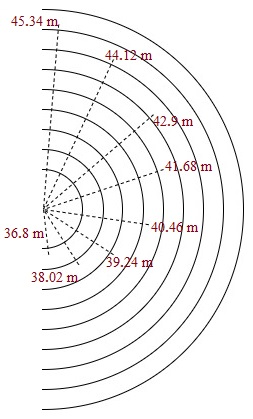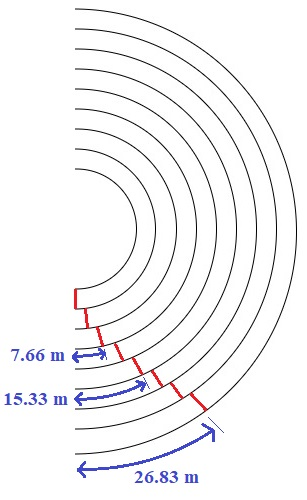### Athletics (200 m and 400 m)

Building on the previous post, I will analyse how my school designed the track for the 200 metre (m) sprint  and 400 m race on Sports Day. The same track was also used for the 4 x 100 m relay events.

# The standard track length

The shortest standard track in athletics is shown below. It is 400 m long when measured along the innermost lane (refer the logic and calculations below Figure 1).Figure 1

There are 8 lanes in this track - however, this number can be increased/decreased depending on the available space on the field. Each lane has a width of 1.22 m and, in calculations, the runner is estimated to be running at a distance of 0.3 m from the inner side of his/her lane.

The 2 straight segments of the track are 84.39 m long each as evident from Figure 1. But, what about the length of the 2 semi-circular segments, each of inner radius 36.5 m?

You may recall from middle school mathematics that the length of the arc of a semicircle is 𝛑 times the radius with the value of 𝛑 being approximately 3.142.

Using the fact that the runner in the innermost lane is estimated to run at a distance of 0.3 m from the inner side of his/her lane, the radius of the semicircle covered by that runner is 36.5 m + 0.3 m = 36.8 m.

Thus, the length run by the runner in the innermost lane is:

The reason that it is not possible to get exactly 400 m through this mathematical calculation is because of 𝛑 being an irrational number.

Going back to the semi-circular segments... here, the runner in the outermost lane would cover a longer distance compared to the runners in any of the other lanes as the radius of his/her semicircular path would be the highest. So, how is the race designed to be fair to all the athletes regardless of his/her lane? Let's explore this in more detail...

# The semi-circular segments

The semi-circular segment of the track (refer Figure 2) with key dimensions labelled is shown below. The radii are marked based on the estimate that the runner runs at a distance of 0.3 m from the inner side of his/her lane. Hence, you will note that the dotted lines extend a little past the arcs to account for the 0.3 m.

Also, recall that the width of each lane is 1.22 m. Thus, each successive radius is 1.22 m longer than the preceding radius.Figure 2 (NOT to scale)

# The distances run

For the 200 m sprint, the runners will cover 1 semicircular segment and 1 straight segment i.e. half the entire track shown in Figure 1. If all the runners started at the red line indicated in Figure 1, then the distances run by each of them would work out as follows:

 200 m sprint     (Table 1) Lane No. Straight segment Semi-circular segment TOTAL ∆ Radius Length 1 84.39 m (this is uniform for all the runners) 36.8 m 115.63 m 200.02 m 0 m 2 38.02 m 119.46 m 203.85 m 3.83 m 3 39.24 m 123.29 m 207.68 m 7.66 m 4 40.46 m 127.13 m 211.52 m 11.5 m 5 41.68 m 130.96 m 215.35 m 15.33 m 6 42.9 m 134.79 m 219.18 m 19.16 m 7 44.12 m 138.63 m 223.02 m 23 m 8 45.34 m 142.46 m 226.85 m 26.83 m

The total distance is obtained by adding the distance covered in the straight segment and the length of the semi-circular segment.

Here, represents the difference between the distance run by any runner and the distance run by the runner in lane 1 (the innermost lane with the smallest radius). This  is the reason why the position of the runners at the start of the race is staggered. An example of the men's 200 m semifinal at the London olympics in 2012 is shown in Figure 3 below. You can see that the runner in a lower numbered inner lane (say, 4) starts behind the runners in the higher numbered outer lanes i.e. 5, 6, 7, 8 and 9.Figure 3 Image source: https://www.flickr.com/photos/marygregory/7746262280

A diagrammatic representation of how these distances are accounted for is shown in Figure 4. The red lines indicate the actual starting positions for each lane.Figure 4 (NOT to scale)

The runner in the last lane (outermost) starts ~26.83 m ahead of the runner in the first lane (innermost) to ensure a fair race with each athlete running 200 m. Compensations along similar lines are made for each of the other lanes. I have labelled the distances for only a few of them to make the diagram less cluttered!

For the 400 m race, the runners will cover the entire track and finish the race back at the starting point. If all the runners started at the red line indicated in Figure 1, then the distances run by each of them would be double those shown in the 200 m sprint table. Thus, the staggered distances will also need to be doubled.

In other words, the runner in lane 8 (outermost) now starts ~53.66 m (twice of 26.83 m) ahead of the runner in the lane 1 (innermost) so that both athletes will run 400 m by the end of the race.

# My school track

The dimensions of the available playground area at my school (as discussed in detail in the previous post) are shown below.

Clearly, the standard 400 m track would not have fitted on my school playground! 😁 The organisers created a track that was 200 m all the way around and reduced it from an 8 lane track to a 6 lane track to fit our grouund. It looked something like this...Figure 6

I have labelled only the dimensions of the outermost boundary of the track for simplicity. The approximate length of the outermost lane can be calculated as follows:

You can see that the length is ~220 m which approximately corresponds to lane 6 in Table 1. Thus, my school was able to adapt the standard track and create a version that fitted in the playground. 400 m races simply became 2 laps of this 200 m track. 4 x 100 m relays could also be easily run on this track.

I wrote these two posts - the one on the 100 m track and this one - to show you the simplicity and beauty of the math involved in the design of the tracks and the role of math in deciding how the races were conducted. The track design is probably something that we assumed to be true when we were in school without questioning the mathematical reasoning behind it. 😃 In hindsight, it would have been wonderful if my school teachers could have brought math to life and taken it beyond the textbook through such realistic examples.

Lastly, the concepts used in these posts (Pythagoras' theorem, semicircles, perimeter, circumference, length/distance etc.) are universally covered in school mathematics and one of my goals was to explain how these concepts came together in a practical scenario. I hope that the posts provided you food for thought; I know I certainly enjoyed researching and writing them! 😀

1.Very good analysis and explained really well. That too mathematically. Kudos to your effort

2.Neat! reminds me also of a classic problem (that I think we encountered in Marcus' class). If you run at a pace of 15km/hr on your first lap, how quickly would you need to run your second lap to make your average speed 30km/hr?

1.Yeah, Graham... I recall this rather 'impossible' problem too! :-)

3.Very beautifully explained :)